Learn all Concepts of Chapter 4 Class 10 (with VIDEOS). Check - Quadratic Equations - Class 10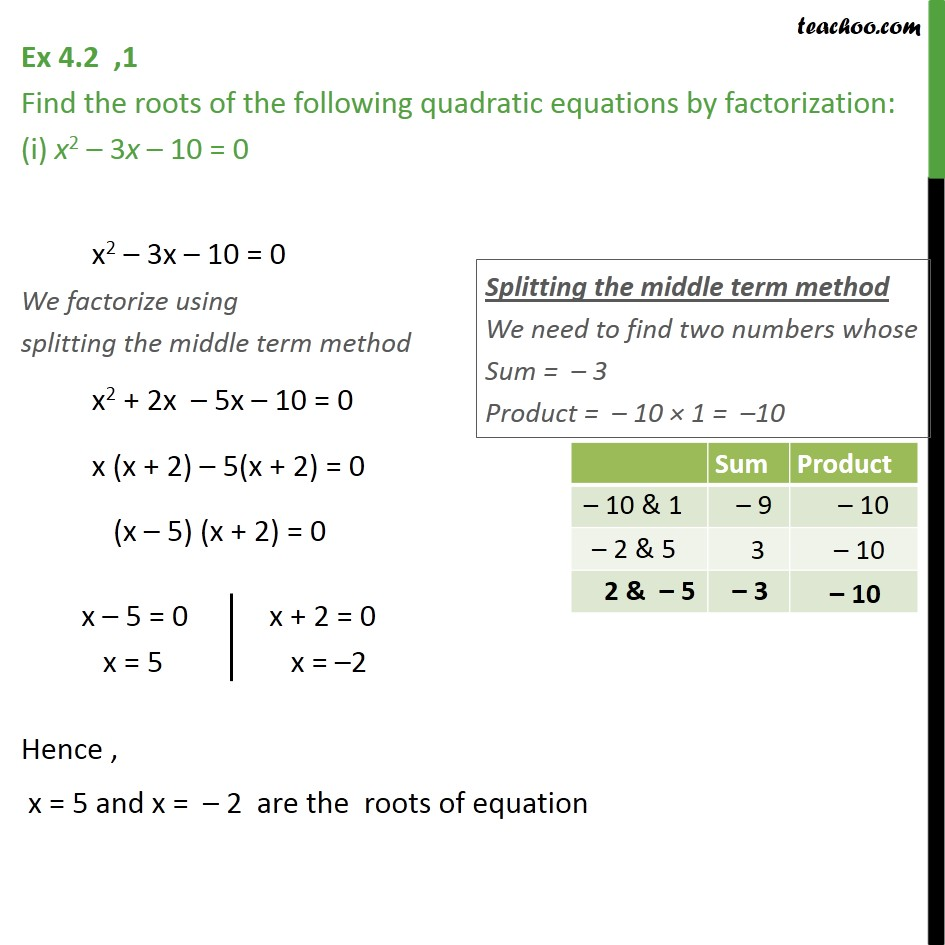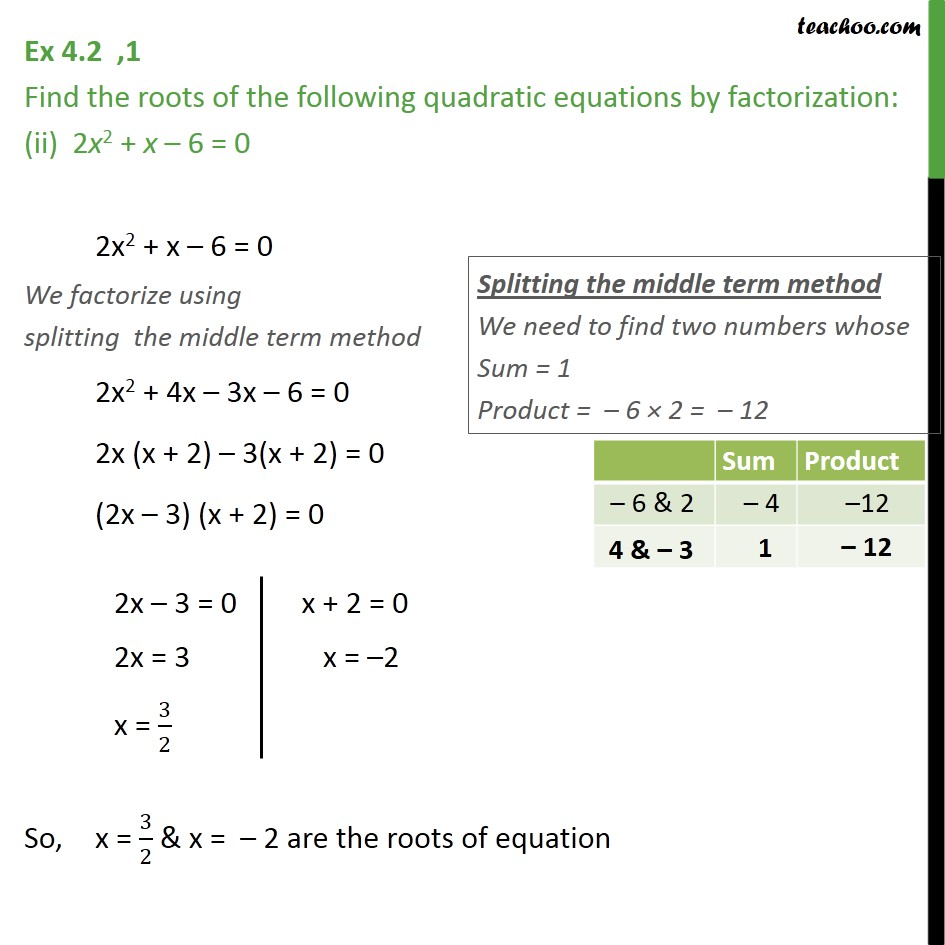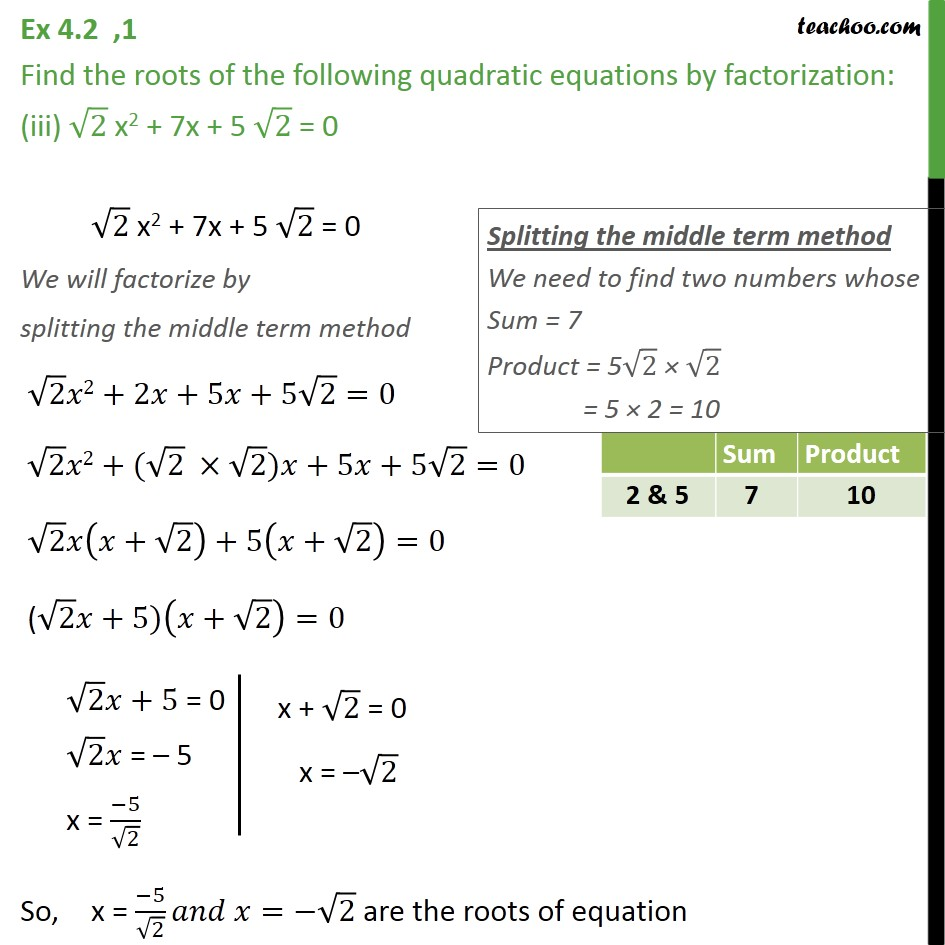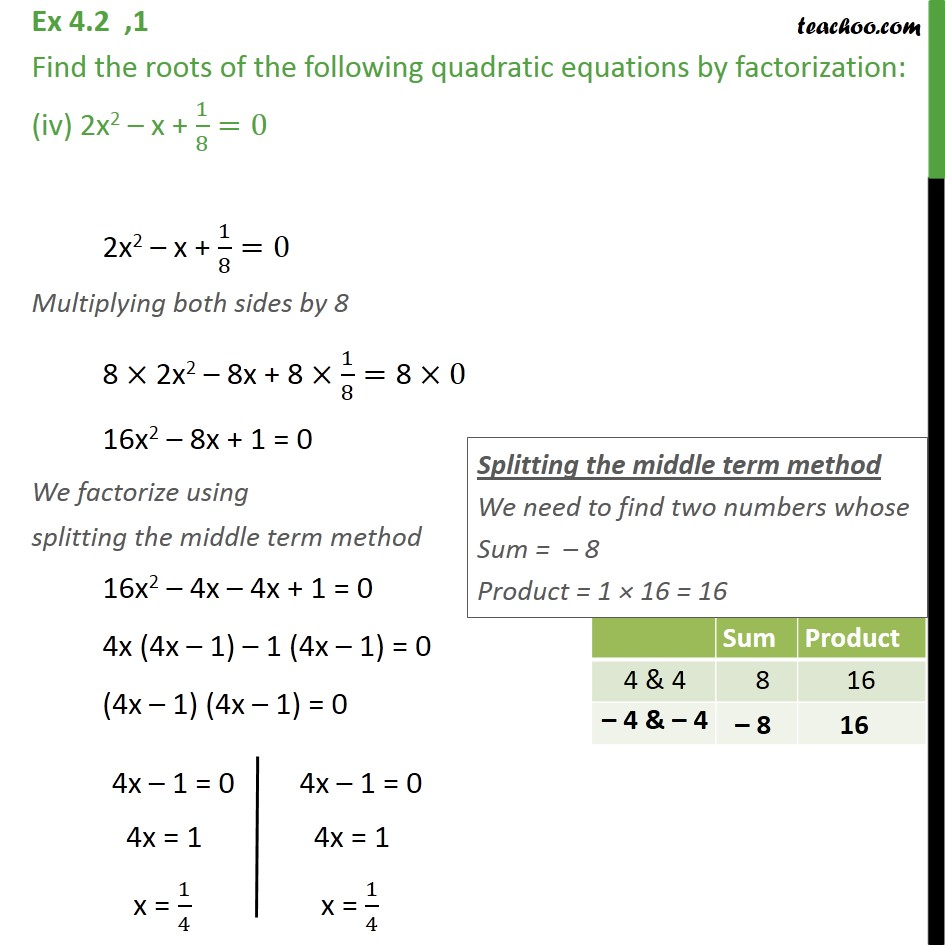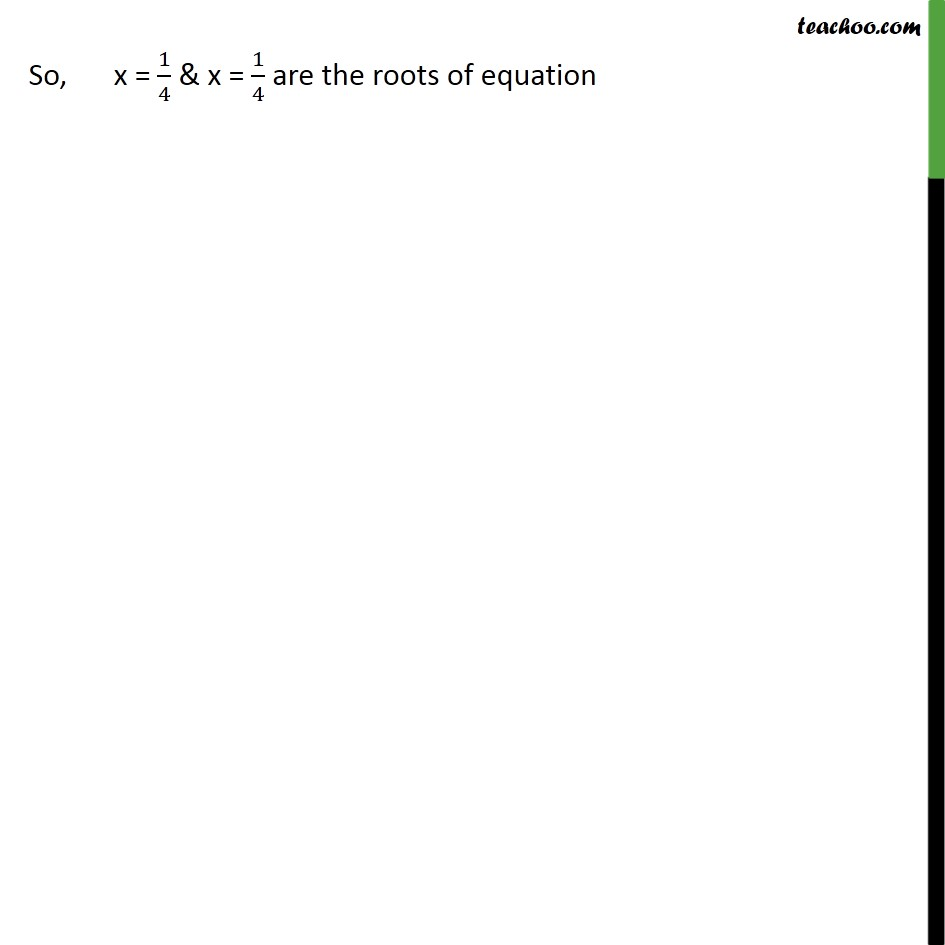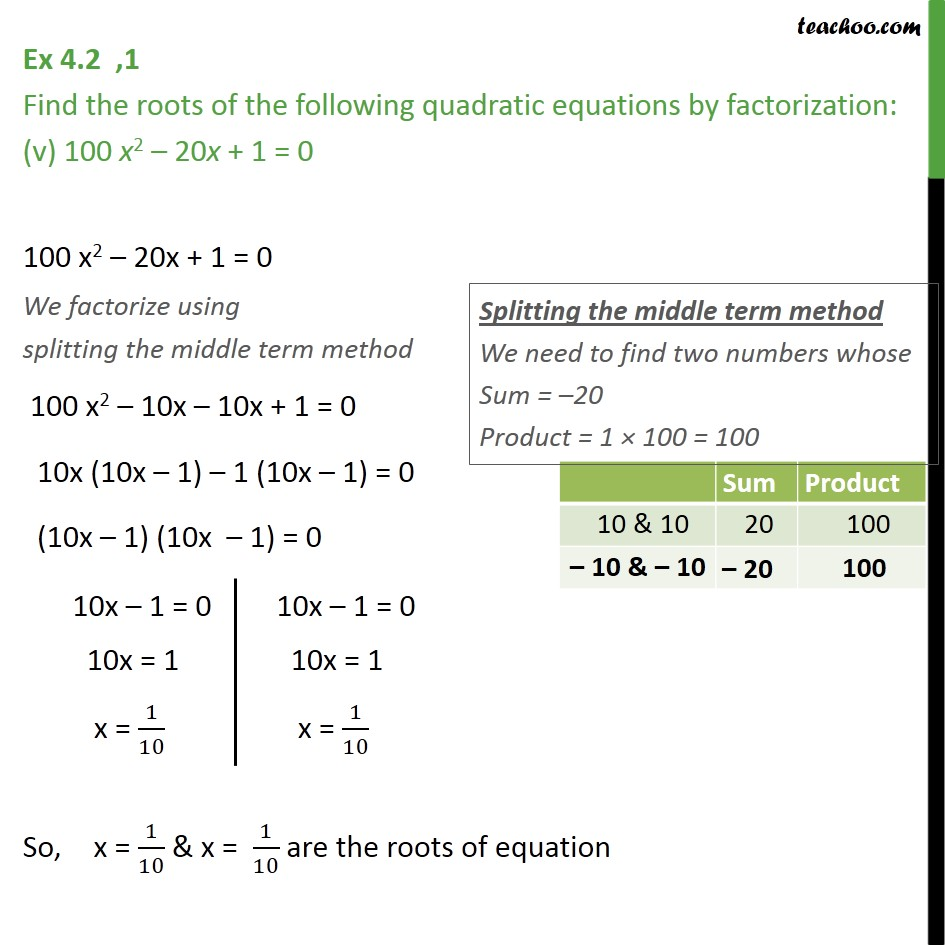1. Chapter 4 Class 10 Quadratic Equations
2. Serial order wise
3. Ex 4.2

Transcript

Ex 4.2 ,1 Find the roots of the following quadratic equations by factorization: (i) x2 3x 10 = 0 x2 3x 10 = 0 We factorize using splitting the middle term method x2 + 2x 5x 10 = 0 x (x + 2) 5(x + 2) = 0 (x 5) (x + 2) = 0 Hence , x = 5 and x = 2 are the roots of equation Ex 4.2 ,1 Find the roots of the following quadratic equations by factorization: (ii) 2x2 + x 6 = 0 2x2 + x 6 = 0 We factorize using splitting the middle term method 2x2 + 4x 3x 6 = 0 2x (x + 2) 3(x + 2) = 0 (2x 3) (x + 2) = 0 So, x = 3/2 & x = 2 are the roots of equation Ex 4.2 ,1 Find the roots of the following quadratic equations by factorization: (iii) 2 x2 + 7x + 5 2 = 0 2 x2 + 7x + 5 2 = 0 We will factorize by splitting the middle term method 2 2+2 +5 +5 2=0 2 2+( 2 2) +5 +5 2=0 2 ( + 2)+5( + 2)=0 ( 2 +5)( + 2)=0 So, x = ( 5)/ 2 = 2 are the roots of equation Ex 4.2 ,1 Find the roots of the following quadratic equations by factorization: (iv) 2x2 x + 1/8=0 2x2 x + 1/8=0 Multiplying both sides by 8 8 2x2 8x + 8 1/8="8 " 0 16x2 8x + 1 = 0 We factorize using splitting the middle term method 16x2 4x 4x + 1 = 0 4x (4x 1) 1 (4x 1) = 0 (4x 1) (4x 1) = 0 So, x = 1/4 & x = 1/4 are the roots of equation Ex 4.2 ,1 Find the roots of the following quadratic equations by factorization: (v) 100 x2 20x + 1 = 0 100 x2 20x + 1 = 0 We factorize using splitting the middle term method 100 x2 10x 10x + 1 = 0 10x (10x 1) 1 (10x 1) = 0 (10x 1) (10x 1) = 0 So, x = 1/10 & x = 1/10 are the roots of equation

Ex 4.2